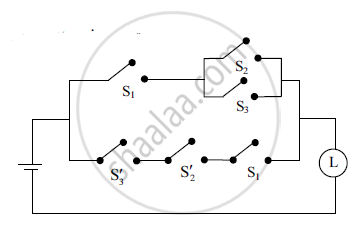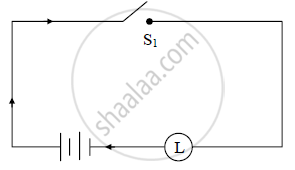HSC Arts 12th Board ExamMaharashtra State Board
Share

# Construct the New Switching Circuit for the Following Circuit with Only One Switch by Simplifying the Given Circuit: - HSC Arts 12th Board Exam - Mathematics and Statistics

ConceptMathematical Logic Application - Introduction to Switching Circuits

#### Question

Construct the new switching circuit for the following circuit with only one switch by simplifying the given circuit:#### Solution

Let p : The switch S1 is closed.

q : The switch S2 is closed.

r : The switch S3 is closed.

~p: The switch 1 S′ is closed or the switch S1 is open.

~q: The switch 2 S′ is closed or the switch S2 is open.

~r : The switch 3 S′ is closed or the switch S3 is open

The logical expression corresponding to the given circuit is

[p ∧ (q ∨ r)] ∨ [~r ∧ ~q ∧ p]

≡ [p ∧ (q ∨ r)] ∨ [~(r ∨ q) ∧ p] ….(De-Morgan’s law)

≡ [p ∧ (q ∨ r)] ∨ [~(q ∨ r) ∧ p] ….(Commutative law)

≡ [p ∧ (q ∨ r)] ∨ [p ∧ ~(q ∨ r)] ….(Commutative law)

≡ p ∧ [(q ∨ r) ∨ ~(q ∨ r)] ….(Distributive law)

≡ p ∧ T ….(Complement law)

≡ p ….(Identity law)

The simplified circuit is asIs there an error in this question or solution?

#### APPEARS IN

2012-2013 (October) (with solutions)
Question 3.1.1 | 3.00 marks
Solution Construct the New Switching Circuit for the Following Circuit with Only One Switch by Simplifying the Given Circuit: Concept: Mathematical Logic - Application - Introduction to Switching Circuits.
S